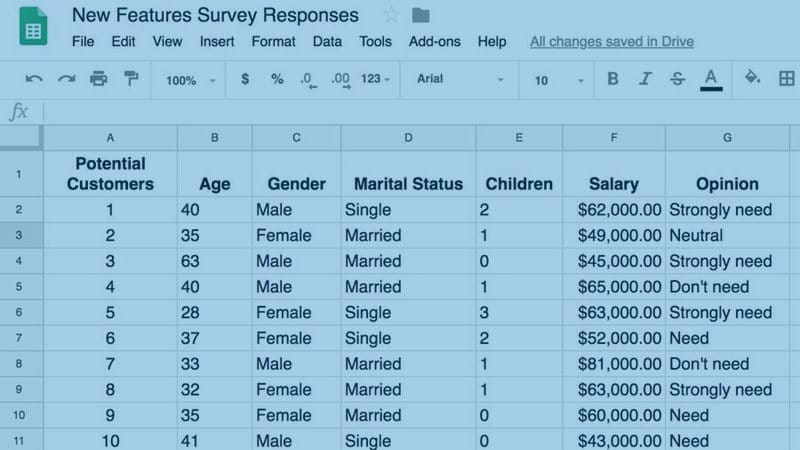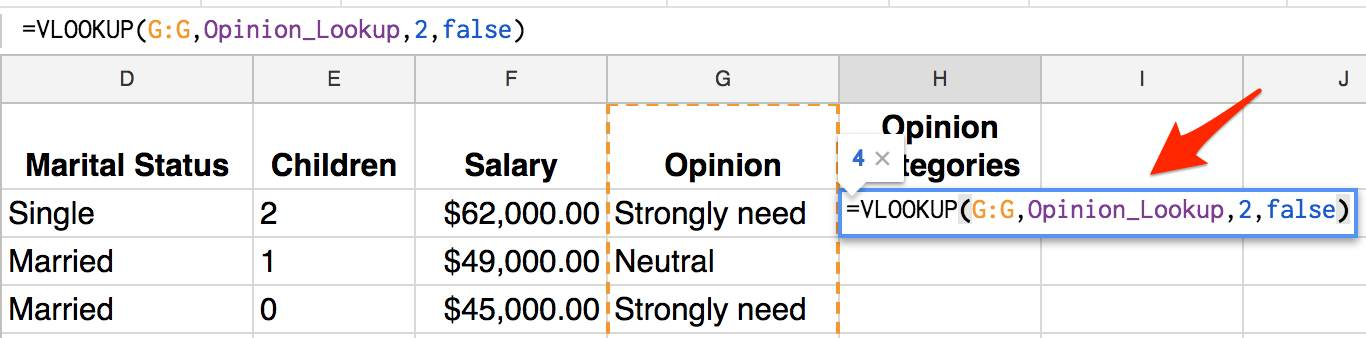# Using VLOOKUP Function in Google SheetsI will start the Analytics category here with this important function for your analytical skills. Many times you will need to play with the data you have to make a different analysis. I will share many tutorials here related to statistics and data analytics. Here we will look at the VLOOKUP function in Google Sheets. This is only the beginning.

In most of the cases, I use the VLOOKUP function to change textual into numerical data, or vice versa. For example, if I have the “Municipality” column in my data, and I want to make some correlation analysis between the size of municipality and other variables I will need to categorize somehow textual record of “Municipality” column. In such a case I will use VLOOKUP function to search in another table with records for the size of each municipality related with categorical value. The lookup table will have the name of municipality and category for 1 – small, 2 – medium and 3-large. This function will search in my original data, find the value from the lookup table and select the category from the lookup table to insert in my new column “Municipality Size.”

Let’s see how we can use this VLOOKUP function in Google Sheets to analyze some recorded data.

### VLOOKUP Function in data from the survey

If you have surveyed with 40 potential customers from different demographics to analyze their need for new product features that your company need to innovate you will get some results like in this table.As you can see we have several columns that can be additionally described in the mathematical language to use some statistical approaches to analyze them. For example, an opinion column can use additional categories to give them some new meaning in the data. For, the opinion we have textual data as:

• Don’t need this feature
• Neutral
• Need
• Strongly need

In such a case if the potential customer doesn’t need this feature, we can use number 1, for neutral 2, need 3 and strongly need 4.

This approach when we categorize the variables is called binning because we put the data into discrete bins. Sometimes it is also called discretizing the data.

#### 1. Create Lookup Tables

So, the first step we need to do in our data is to define our lookup tables for these three variables. I will make on the side on my original data three lookup tables: Opinions, Age, and salary lookup.#### 2. Create a Named Range in Google Sheets

Next thing we need to do after the creation of lookup tables is to create a named range in Google Sheets, so it will be much easier to use them when we execute VLOOKUP function.

To create a named range, we will need to select the table (highlight the range), and with right-click, you need to select “Define named range…”.When we click on the “Define named range…” on the right side will appear sidebar where we will need to write the name of the range. In this case, I choose “Opinion_Lookup” to be the name of the range.#### 3. Execute VLOOKUP Function

Now we have all things prepared, and we can quickly execute the VLOOKUP function to get three new columns with the categorized data.

As a first, you will need to add one new column to the right of your initial data and name it as “Opinion Categories.” Now in the first row for data in this new column, you will need to start writing “=VLOOKUP” and the function will appear explaining what you need to select.

We will use the following formula to find category values for potential customer’s opinion “=VLOOKUP(G:G,Opinion_Lookup,2,false)”.• The first thing we need to select is the search_key that represents the value to search for. In our case, we want to search in Opinion Column (G:G) for values.
• The second part is the range to consider for the search. So, the function will search in the first column in the range for the key specified in search_key.
• Next part is the index or column index of the value to be returned. In our case, we want to return the data from column 2 in the Opinion_Lookup table.
• The last part is “is_sorted” that asks whether the column to be searched is sorted. In our example, we will use the false.

When you hit the enter button, the returned value will be four that means “Strongly need.” If we copy this data from cell H2 and paste it in the rest of the column (H3: H41), we will get categorized values for all data.Probably you think why you need to do these steps when you can easily add numbers one by one. But, take into consideration that here we look at 40 data records. What if we have several thousand records? How much time will you need to do this? So, this is a beneficial function when you analyze massive amounts of data.